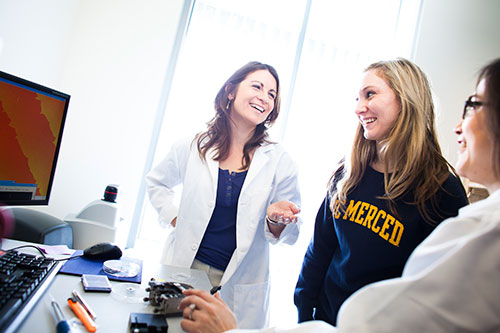# Applied Mathematical Sciences, B.S.

##Overview of Applied Mathematical Sciences

Mathematics has been a central feature of humanity's intellectual achievements over the past several centuries. Its role in the physical sciences and engineering is well established and continues to aid in their development. However, mathematics is becoming more important in the social sciences and life sciences which are all new application areas for applied mathematical sciences. Thus, the field is undergoing remarkable growth.

UC Merced offers an undergraduate major leading to a B.S. degree in the Applied Mathematical Sciences. This educational experience provides the foundations of mathematics and the skills needed to apply mathematics to real-world phenomena in the social sciences, natural sciences and engineering. The curriculum is designed to provide courses in the fundamentals while allowing for building expertise in an application area through the emphasis tracks. There is a core set of courses all mathematical sciences students take. Beyond those courses, students take an emphasis track consisting of courses in other fields. New emphasis tracks will be added along side new programs developing at UC Merced. Students may also design their own emphasis track with the approval of the faculty program leads for the Applied Mathematical Sciences major.

There are seven emphasis tracks within the Applied Mathematical Sciences major:

• Computational and Data Sciences
• Computational Biology
• Computer Science
• Economics
• Engineering
• Environmental
• Physics

###Areas of Research

• Asymptotic analysis and perturbation methods
• Dynamics and design problems for circuits and electromagnetic media
• Image processing and compressed sensing
• Ultrafast laser and nonlinear optics

### Potential Careers

• Actuary
• Fluid dynamics expert
• Mathematical physicist
• Statistician
• Teacher or professor
• Financial analyst
• Theoretical mathematician
• Computer systems analyst
• Computer programmer
• Biostatistician

* Please Note: Some of these carers might require education beyond a Bachelor's degree.

### Learning Outcomes

Students graduating from the Applied Mathematical Sciences program demonstrate the following:

The over-arching goal of the Applied Mathematical Sciences program is to build a community of life-long learners that use the analytical and computational tools of mathematics to solve real world problems.

Upon graduating, we expect students completing the Applied Mathematical Sciences major to have become effective problem solvers, meaning that student will be able to

1. Solve mathematical problems using analytical methods.
2. Solve mathematical problems using computational methods.
3. Recognize the relationships between different areas of mathematics and the connections between mathematics and other disciplines.
4. Give clear and organized written and verbal explanations of mathematical ideas to a variety of audiences.
5. Model real-world problems mathematically and analyze those models using their mastery of the core concepts.

Sample plans of study for the Applied Mathematical Sciences degree may be found in the School of Natural Sciences website ns-advising.ucmerced.edu.